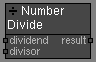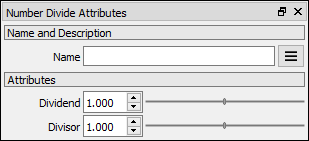# Number Divide node

Left Toolbar >Math Nodes >Number Divide

The Number Divide node allows you to execute a division operation of two Number values.

## Procedure

### To execute a division operation on two Node Values in the node graph:

1. On the left toolbar choose Math Nodes> Number Divide2. Drag the Number Divide icon into the Node Graph.3. Connect the Output: result.

## Inputs

dividend A NUMBER type of value.

divisor A NUMBER type of value.

## Outputs

result The number value. result = divident / divisor.

## Attributes PanelDividend The first term in the division operation. This attribute is exposed as input by default.

Divisor The second term in the division operation. This attribute is exposed as input by default.

Math Nodes

Main Page